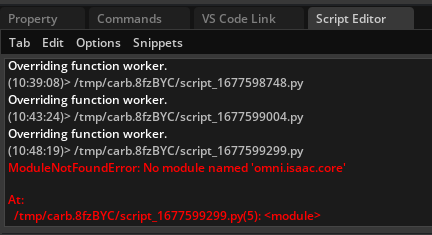# How can I force the camera to look at the object(s) of interest and take photos of them when randomizing positions?

I have the following code which basically just randomizes the position of a worker inside a plane.

``````import omni.replicator.core as rep
from datetime import datetime
import omni.usd

# import omni.isaac.core.utils.bounds as bounds_utils
# import omni.isaac.core.utils.prims as prims_utils

with rep.new_layer():
# Define paths for the character, the props, the environment and the surface where the assets will be scattered in.

WORKER = 'omniverse://localhost/NVIDIA/Assets/Characters/Reallusion/Worker/Worker.usd'

plane = rep.create.plane(scale=100, visible=True, semantics=[("class", "plane")])

print('type of plane: ', print(plane))

# bb_cache = bounds_utils.create_bbox_cache()
# combined_range_arr = bounds_utils.compute_combined_aabb(bb_cache, prim_paths=plane)
# print('combined_range_arr: ', combined_range_arr)

def worker():
worker = rep.create.from_usd(WORKER, semantics=[('class', 'worker')])

with worker:
rep.modify.pose(
position=rep.distribution.uniform((-1000, 0, -1000), (1000, 0, 1000)),
rotation=rep.distribution.uniform((-90,-45, 0), (-90, 45, 0)),
)
return worker

# Register randomization

rep.randomizer.register(worker)

# Setup camera and attach it to render product
camera = rep.create.camera(
focus_distance=800,
f_stop=0.5
)
render_product = rep.create.render_product(camera, resolution=(1024, 1024))

timestamp = datetime.now().strftime("%Y_%m_%d-%I_%M_%S_%p")
# Initialize and attach writer
writer = rep.WriterRegistry.get("BasicWriter")
writer.initialize(output_dir="/home/mona/Desktop/Isaac_Sim_Dummy_Out/vegetation/" + timestamp , rgb=True, bounding_box_2d_tight=True)
writer.attach([render_product])

with rep.trigger.on_frame(num_frames=100):

rep.randomizer.worker()
with camera:
rep.modify.pose(position=rep.distribution.uniform((-500, 200, 1000), (500, 500, 1500)), look_at=plane)
``````

Here, if I change the look_at to worker it gonna throw an error since worker is a function.

Also, given the current code, there are naturally situations when the camera only takes photos of the plane and not the worker.

So,

1. if I have one object of interest, how can I force a camera with randomized position to always look the the object of interest?
2. if I have multiple objects of interest, how can I force a camera with randomized position to always look at the mean pose of objects of interest or something similar?

I am using Omniverse Code Replicator and dumped the code in Script Editor, hit Run and then clicked on Replicator → Start.

For example, rgb_0099.png is not capturing the object of interest, here, the worker.

Also, how can I get the bounding box of an object, here the plane? I don’t have access to functions from Isaac Sim inside Omniverse Code @toni.smFurther, why is type of plane is stated as None? Isn’t a prim? I intend to pass the plane to `compute_combined_aabb` like below:

``````    bb_cache = bounds_utils.create_bbox_cache()
combined_range_arr = bounds_utils.compute_combined_aabb(bb_cache, prim_paths=plane)
print('combined_range_arr: ', combined_range_arr)
``````

The goal of finding the bbox is to ensure the worker is not spawn outside of plane.

Actually, got the look_at work but @toni.sm please let me know if there is an alternative for getting bounding box in Omniverse Code Script Editor.

``````import omni.replicator.core as rep
from datetime import datetime
import omni.usd

# import omni.isaac.core.utils.bounds as bounds_utils
# import omni.isaac.core.utils.prims as prims_utils

with rep.new_layer():
# Define paths for the character, the props, the environment and the surface where the assets will be scattered in.

WORKER = 'omniverse://localhost/NVIDIA/Assets/Characters/Reallusion/Worker/Worker.usd'

plane = rep.create.plane(scale=25, visible=True, semantics=[("class", "plane")])

print('type of plane: ', print(plane))

# bb_cache = bounds_utils.create_bbox_cache()
# combined_range_arr = bounds_utils.compute_combined_aabb(bb_cache, prim_paths=plane)
# print('combined_range_arr: ', combined_range_arr)

def randomize_worker():

worker = rep.create.from_usd(WORKER, semantics=[('class', 'worker')])
with worker:
rep.modify.pose(
position=rep.distribution.uniform((-1000, 0, -1000), (1000, 0, 1000)),
rotation=rep.distribution.uniform((-90,-45, 0), (-90, 45, 0)),
)
return worker

# Register randomization

rep.randomizer.register(randomize_worker)

# Setup camera and attach it to render product
camera = rep.create.camera(
focus_distance=800,
f_stop=0.5
)
render_product = rep.create.render_product(camera, resolution=(1024, 1024))

timestamp = datetime.now().strftime("%Y_%m_%d-%I_%M_%S_%p")
# Initialize and attach writer
writer = rep.WriterRegistry.get("BasicWriter")
writer.initialize(output_dir="/home/mona/Desktop/Isaac_Sim_Dummy_Out/vegetation/" + timestamp , rgb=True, bounding_box_2d_tight=True)
writer.attach([render_product])

with rep.trigger.on_frame(num_frames=100):

worker = rep.randomizer.randomize_worker()
with camera:
rep.modify.pose(position=rep.distribution.uniform((-1000, 200, 1500), (1000, 400, 2000)), look_at=worker)
``````

This topic was automatically closed 14 days after the last reply. New replies are no longer allowed.